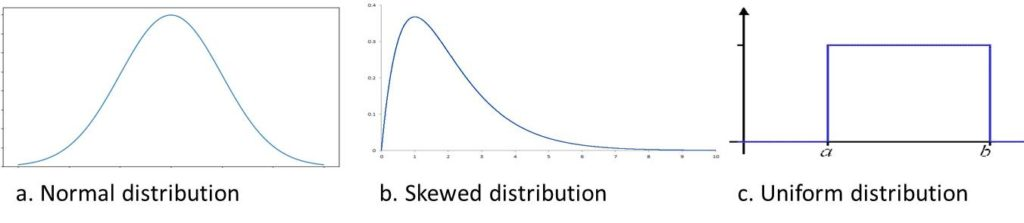# Statistics Tutorial 1 – Introduction to SamplingThis is the very first lesson in Statistics Tutorial Series.

We would be discussing Introduction to Sampling and Sampling Distributions.

First, let define the two basic terms: Population and Sample

What is a Population?

Population is the entire set of entities or individual we want to study. For example, the population of students in West Africa. Another example is population of all birds in Australia

What is a Sample?

A sample is a subset of the population we are researching on. For example, 50 bird collected from Australia.So the idea is to use this sample to generalize about the population since it’s not practical to access all members of a population.

#### What is Sampling Distribution?

This has to do with taking repeated samples but is clearer if we use an example.

Assuming we want to carry out a study on students in Nigeria. Then we can take random samples from different cities.

For example, we take sample of 20 students from Enugu, then we take another sample of 20 students from Lagos, then another 20 students from Port Harcourt and so on. This means that  we end up with several samples of 20 students per sample. So what we would have is a sample distribution. This is because these samples are different, that is distributed across the population.

Type of Distribution

I would just mention three types of distributions.They are normal, skewed and uniform distribution as shown in Figure 1.

So take for instance, you take so many samples from the population ( say all possible samples). Then you calculated the average(maybe average age of each student) of each of these samples. Then you plot these averages in a histogram, you will have a shape like shown in the Figure 1 a, b or c.Figure 1: Possible Sampling Distributions

Just to repeat, if we calculated the mean of every sample,  then we get a ‘Sampling Distribution of Sample Means’.

Although there other types of sampling distribution, we would mostly be talking about sampling distribution of sample means.

Finally, note that sample size is normally represented with n.

In the next lesson, we would discuss Central Limit Theorem.